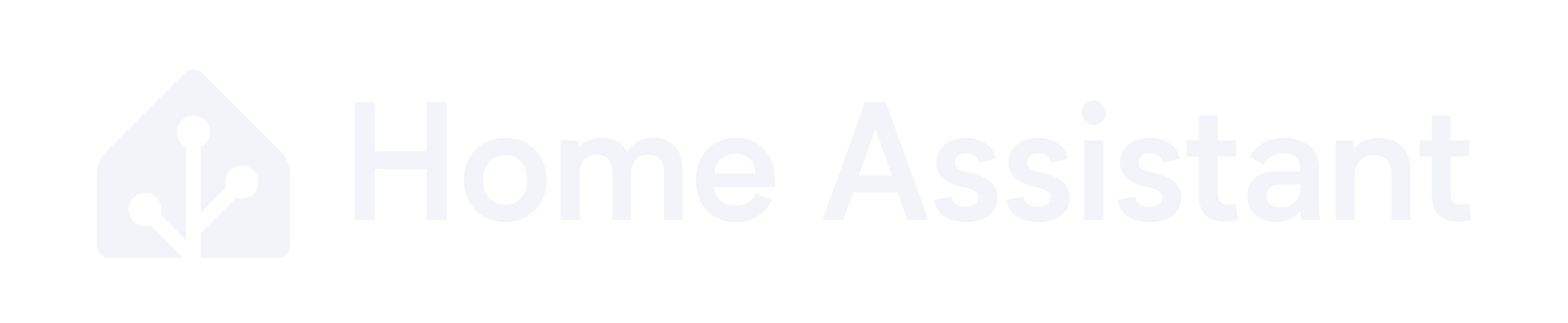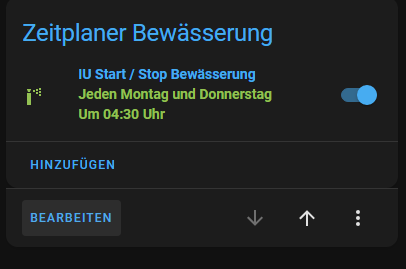# Add runtime to a given time?

Hi everyone,

How can I create a template sensor by taking the following 2 criteria into account.
I will know the end times how long the irrigation will run.
I have a scheduler card where watering starts at 4:30 am and a sensor with the total watering time.
So the watering ends at 6:30 a.m.
I want to combine the two things``````{% set some_datetime = now() %}
{{ some_datetime + timedelta(minutes=42) }}
``````

Can use the attributes of scheduler?
For example timeslots or next_trigger?
If yes how?

What have you tried?

Nothing yet.
Hence my question…

See the intro and #9.

Hi,

so far i figured that out.
But the value is not updated.

``````{{ state_attr('switch.schedule_0ce9ef', 'next_trigger') | as_datetime + timedelta(minutes = states('sensor.zone_gesamt_time_total')|int(0)) }}
``````

Ok good, now we can try to get somewhere.

What is the output of this?

``````{{ state_attr('switch.schedule_0ce9ef', 'next_trigger') | as_datetime }}
{{ states('sensor.zone_gesamt_time_total') }}
``````

Do you mean the output as you see it in the dev tools don’t have the timedelta applied?

Are you expecting it to update the `next_trigger`? If yes, that’s not how templates work. They do not set values.

Yes, that’s what I mean.
Watering begins at 4:30 a.m.
At 6:40 a.m. the watering is finished.
I need this value (6:40 a.m.)

So 4:30 am + 2.10 hours = 6:40 am

Then you’re just getting the default back from this entity. What’s that entities state?

Exactly what I asked for too, but not given, so who knows.

I think you’ve nailed it. This is probably the (incorrect) expectation.

@Kabala sensor values are immutable in templates. You can assign the result to a variable or use the output of a template to set a template sensor’s value, but you can’t change the existing value in place.

Maybe we can get further with the template of sensor.zone_gesamt_time_total.
Is that possible?

``````platform: template
sensors:
zone_gesamt_time_total:
friendly_name: 'Gesamtzeit '
icon_template: 'mdi:clock-start'
value_template: >
{% set update = states('sensor.time') %}
{% set ns = namespace(values=[]) %}
{% for i in expand('group.zone_gesamt') | list %}
{% set ns.values = ns.values + [ states('input_number.'~i.object_id~'')|int ] %}
{% endfor %}
{% set t =  ns.values | sum %}
{{ '{}h {}min'.format(t//60, t%60) }}
``````

As per a previous reply, paste this, and exactly this.

``````{{ state_attr('switch.schedule_0ce9ef', 'next_trigger') | as_datetime }}
{{ states('sensor.zone_gesamt_time_total') }}
``````

2h 0min is not an integer, so you can’t convert it to an integer. This will get complicated if the format changes based on the time. To be honest, tha’ts not a normally formatted attribute for a time and because of this it makes it harder than normal to use.

``````{% set t = state_attr('switch.schedule_0ce9ef', 'next_trigger') | as_datetime %}
{% set h, m = states('sensor.zone_gesamt_time_total') | regex_findall('^(?:(?P<h>[0-9]{1,2})h ){0,1}(?:(?P<m>[0-9]{1,2})min){0,1}') | first | default(['','']) | map('float', 0) %}
{{ t + timedelta(hours=h, minutes=m) }}
``````

I would consider rebuilding the template for `sensor.zone_gesamt_time_total`. It can just sum seconds and output that, with the appropriate device class and UoM. Everything else will then be simpler, including using it with the `timedelta` function.

hi Petro,
thanks for the configuration, works very well.
I still have two questions.
How can I display the date format in iso?dd/mm/yyyy
And the Coordinated Universal Time ( UTC ) how can I hide that?

It’s already output in iso format, which is yyyy-mm-dd. If you want it output in a different format than that, you can use `.strftime`

``````{% set t = state_attr('switch.schedule_0ce9ef', 'next_trigger') | as_datetime %}
{% set h, m = states('sensor.zone_gesamt_time_total') | regex_findall('^(?:(?P<h>[0-9]{1,2})h ){0,1}(?:(?P<m>[0-9]{1,2})min){0,1}') | first | default(['','']) | map('float', 0) %}
{{ (t + timedelta(hours=h, minutes=m)).strftime('%d/%m/%Y %H:%M:%S') }}
``````

Just keep in mind that all these format changes will make these entities harder to use in automations. If you simply stick to normal formats, with typical sensors in HA, the frontend will translate all times into your selected format.

@parautenbach is on the correct path saying that you should rework all your template entities.